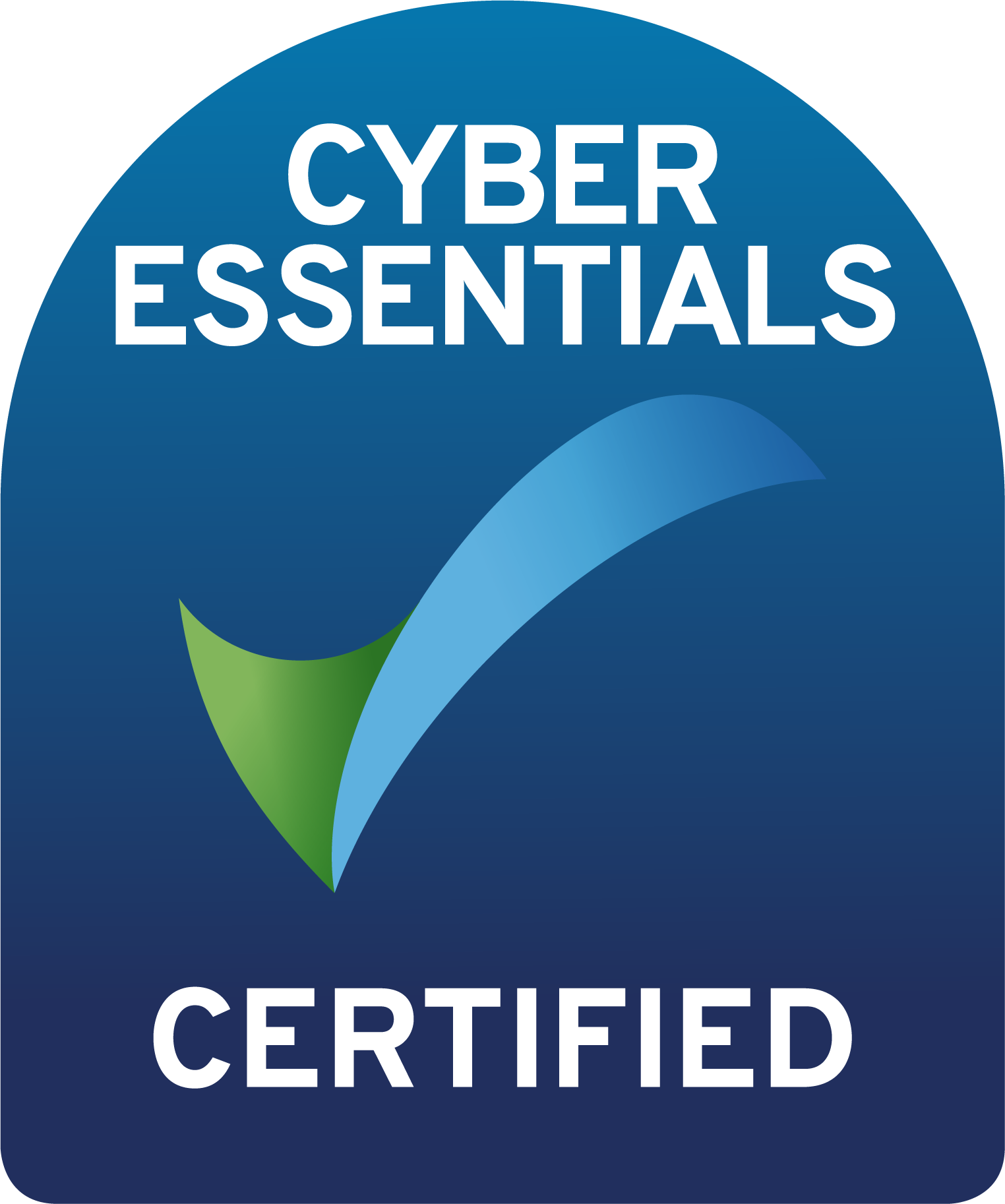# Top 7 most used Excel Functions

As any seasoned Excel user will know, entering the data is only one part of the job, the next step is making that data speak so that you can make criticial business decisions based on the data input. We have compiled here the 7 most used Excel functions you will defintely want to remember.

1) VLOOKUP

Use VLOOKUP to look up and retrieve data from a table.

The Syntax for VLOOKUP is: VLOOKUP (lookup_value, table_array, col_index_num, [range_lookup])

2) IF

Use IF to make comparisons between a value and what you expect

The Syntax for the IF function is: IF(logical_test, value_if_true, [value_if_false])

3) SUM

Use SUM to add up a range of numbers

The Syntax for the SUM function is: SUM(range to be summed)

4) COUNTIF

Use COUNTIF to count the number of cells that meet a criteria

The Syntax for the COUNTIF function is: COUNTIF(range, criteria)

5) SUMIF

Use SUMIF to sum the values in a range that meet a specific criteria.

The Syntax for the SUMIF function is: SUMIF(range, criteria, [sum_range])

6) CONCATENATE

Use CONCATENATE to join two or more text strings into one string.

The Syntax for the CONCATENATE function is: CONCATENATE(text1, [text2], …) To add spaces or punctuation, type the item inquotation marks between the cells or values you want to connect.

7) AVERAGE

Use AVERAGE to retunr the average of a range of numbers.

The Syntax for the AVERAGE function is: AVERAGE (number1, [number2], …)